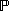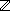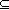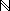### ELEMENTS OF Z

Based on chapter 8 in The Way of Z.

Links to more Z lectures.

This page looks best when thisand this X are about the same size:X. See these viewing tips.

Three basic constructs:

Declarations introduce variables.

Expressions describe values that variables can assume.

Predicates place constraints on the values that variables do assume.

SETS

Set display

 { 1, 2, 3, 4, 5, 6 } { 4, 5, 6, 1, 2, 3 } { 4, 5, 5, 6, 1, 1, 1, 2, 3 } { red, yellow, green } { yellow, red, green, 1, 2 } Type error!

Set namesDICE LAMP

Set expressions

 1 .. 6 {  i:| 1i6  }

TYPES

Every object belongs to a set called its type.

1, 2, ... all belong to the type.

red, green belong to the type COLOR.

Every type must be introduced in a declaration. There are two ways to declare types.

Free types are like enumerations.

 COLOR ::= red | green | blue | yellow| cyan | magenta | white | black

Basic types can include indefinitely many elements.[NAME] [PROCESS, FILE]

VARIABLES

A variable is a name for an object: its value. Variables are introduced in declarations.

 x: S     The value of x belongs to set S

Axiomatic definitions declare global variables and can include optional constraints.d1,d2: DICEd1 + d2 = 7 d1 < d2Constants are variables that are constrained to one value (S means set of S).DICE:DICE = 1 .. 6Abbreviation definitions can also declare constants.

 DICE == 1 .. 6

TYPES, SETS AND NORMALIZATION

Types are sets, but not all sets are types.

ODD, EVEN, PRIME are just sets,is their type.

Any set can appear in a declaration.e: EVEN o: ODD p: PRIMEIn a normalized declaration, we write the signature to show the type.e,o,p:eEVEN oODD pPRIMEThe type determines which variables can be combined in expressions.

EXPRESSIONS AND OPERATORS, ARITHMETIC

Expressions have values. The simplest expressions are constants and variables.

 1, 2, red, x, d1, DICE,, ...

Operators build larger expressions from smaller ones. Arithmetic provides familiar examples.

 m+n Addition m-n Subtraction m*n Multiplication m div n Division m mod n Remainder (modulus) mn Less than or equal m .. n Number range (up to) min A Minimum of a set of numbers max A Maximum of a set of numbers

SET OPERATORS

The size operator # counts elements.

 # { red, yellow, blue, green, red } = 4

The union operatorcombines sets.

 { 1, 2, 3 }{ 2, 3, 4 } = { 1, 2, 3, 4 }

The difference operator \ removes the elements of one set from another.

 { 1, 2, 3, 4 } \ { 2, 3 } = { 1, 4 }

The intersection operatorfinds the elements common to both sets.

 { 1, 2, 3 }{ 2, 3, 4 } = { 2, 3 }

Set operators work with sets of any type, but

{ 1, 2, 3 }{ red, green }     Type error!

PREDICATES

Predicates constrain values. Many have the form e1 R e2, where e1 and e2 are expressions.

Equality, x and y have the same value.

 x = y

Arithmetic relations, n is less than m.

 n < m

Set membership, x is a member of S.

 xS

Subset, members of S are members of T.

 ST

Predicates are not expressions. They do not have values, they are true or false.

PUTTING THE ELEMENTS TOGETHER

A train moves at a constant velocity of sixty miles per hour for four hours.distance, velocity, time:distance = velocity * time velocity = 60 time = 4How far does the train travel?

Philip works on the adhesives team in the materials group, which is part of the research division.philip: PERSON adhesives, materials, research,manufacturing:PERSONadhesivesmaterials materialsresearch philipadhesivesIs Philip in the research division?

ELEMENTS OF Z: DISCUSSION

1. Compare this Z

 DICE == 1 .. 6d1,d2: DICEd1 + d2 = 7 d1 < d2with this C.

typedef int DICE;
DICE d1, d2;

2. Compare this basic type and definition

 [X]x,y: Xwith this free type.

 X ::= x | y

Back to Z lectures.

Jonathan Jacky / University of Washington / Seattle, Washington / USA
E-mail: jon@u.washington.edu